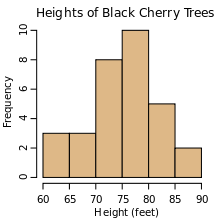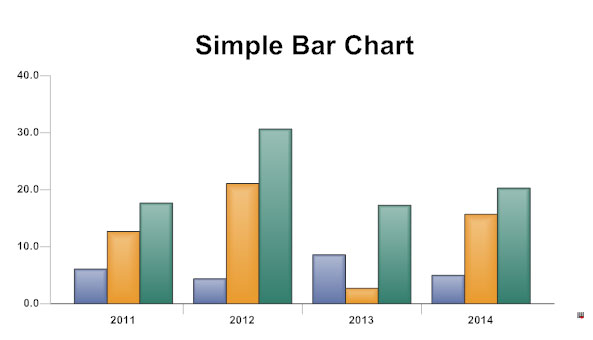# Graphical Representation of Frequency Distribution

Graphical representation can be used for both the educated section and uneducated section of the society. Furthermore, any hidden trend present in the given data can be noticed only in this mode of representation.

We are going to consider the following types of graphical representation

1. Line diagram
2. Histogram
3. Bar diagram
4. Pie chart
5. Frequency polygon
6. Ogives or Cumulative frequency graphs

1.Line diagram

When the time series exhibit a wide range of fluctuations, we may think of logarithmic or ratio chart where “Log y” and not “y” is plotted against “t”.We use Multiple line chart for representing two or more related time series data expressed in the same unit and multiple – axis chart in somewhat similar situations, if the variables are expressed in different units.

1. Histogram

A two dimensional graphical representation of a continuous frequency distribution is called a histogram.In histogram, the bars are placed continuously side by side with no gap between adjacent bars.

That is, in histogram rectangles are erected on the class intervals of the distribution. The areas of rectangle are proportional to the frequencies.

1. Bar diagram

There are two types of bar diagrams namely, Horizontal Bar diagram and Vertical bar diagram.While horizontal bar diagram is used for qualitative data or data varying over space, the vertical bar diagram is associated with quantitative data or time series data.

Bars i.e. rectangles of equal width and usually of varying lengths are drawn either horizontally or vertically.

We consider Multiple or Grouped Bar diagrams to compare related series. Component or sub-divided Bar diagrams are applied for representing data divided into a number of components. Finally, we use Divided Bar charts or Percentage

Bar diagrams for comparing different components of a variable and also the relating of the components to the whole. For this situation, we may also use Pie chart or Pie diagram or circle diagram.

1. Pie chart

In a pie chart, the various observations or components are represented by the sectors of a circle and the whole circle represents the sum of the value of all the components .Clearly, the total angle of 360° at the center of the circle is divided according to the values of the components .

The central angle of a component is =  [ Value of the component  /  Total value] x 360°

Sometimes, the value of the components are expressed in percentages. In such cases,

The central angle of a component is =  [ Percentage value of the component  /  100 ] x 360°

1. Frequency polygon

Frequency Polygon is another method of representing frequency distribution graphically.

Obtain the frequency distribution and compute the mid points of each class interval.

Represent the mid points along the X-axis and the frequencies along the Y-axis.

Plot the points corresponding to the frequency at each mid point.

Join these points, by straight lines in order.

To complete the polygon join the point at each end immediately to the lower or higher class marks (as the case may be at zero frequency) on the X-axis.

1. Ogives or Cumulative frequency graphs

By plotting cumulative frequency against the respective class boundary, we get ogives.

As such there are two ogives – less than type ogives, obtained by taking less than cumulative frequency on the vertical axis and more than type ogives by plotting more than type cumulative frequency on the vertical axis and thereafter joining the plotted points successively by line segments.

## 2 thoughts on “Graphical Representation of Frequency Distribution”

error: Content is protected !!